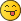Options

# Calculate the resistance of aluminium cable of length 1o km and diameter 2mm

• #### Index

• Calculate the resistance of aluminium cable of length 1o km and diameter 2mm
• ### Calculate the resistance of aluminium cable of length 1o km and diameter 2mm

alielvetaifY > 01-13-2020, 08:10 AM

if the resistivity of aluminium is 2.7×10-8 ΩmLet ρ be the resistivity of the aluminum cable, A the cross sectional area of the cable and l the cable\'s length. The resistance R of the aluminum cable is now given by: R = ρ·l / A = 2.7·10^-8·(10·10^3) / (π·r²) = 2.7·10^-8·(10·10^3) / (π·(1·10^-3)²) = 85.9 Ω I guess the tricky part is remembering: R = ρ·l / A but when you realize how intuitive this equation is you don\'t have to memorize it. The resistance R is proportional to the resistivity ρ, so we must have the following in our equation: R = ρ·k , where k is some value governed by the dimensions of the cable! It is logical that the resistance is also proportional to the wires length l. This is logical when you realize that the longer the wire the more obstacles the electrons will have to pass by! Now we have that: R = ρ·l·c , where c is another value governed now by the cross sectional area (only dimensional property left). If we think of the wire as a highway with cars instead of electrons we realize that there is less resistance (higher speed) when the highway has many lanes and is very broad. From this we realize that the greater the cross sectional area the smaller the resistance. R must therefore be inversely proportional to A. We now have: R = ρ·l / A I hope there are no mistakes hereOther related question